GeeksforGeeks App
Open AppBrowser
Continue

## Related Articles

• Write an Interview Experience
• MongoDB Tutorial

# Aggregation in MongoDB

In MongoDB, aggregation operations process the data records/documents and return computed results. It collects values from various documents and groups them together and then performs different types of operations on that grouped data like sum, average, minimum, maximum, etc to return a computed result. It is similar to the aggregate function of SQL.

MongoDB provides three ways to perform aggregation

• Aggregation pipeline
• Map-reduce function
• Single-purpose aggregation

## Aggregation pipeline

In MongoDB, the aggregation pipeline consists of stages and each stage transforms the document. Or in other words, the aggregation pipeline is a multi-stage pipeline, so in each state, the documents taken as input and produce the resultant set of documents now in the next stage(id available) the resultant documents taken as input and produce output, this process is going on till the last stage. The basic pipeline stages provide filters that will perform like queries and the document transformation modifies the resultant document and the other pipeline provides tools for grouping and sorting documents. You can also use the aggregation pipeline in sharded collection.

Let us discuss the aggregation pipeline with the help of an example: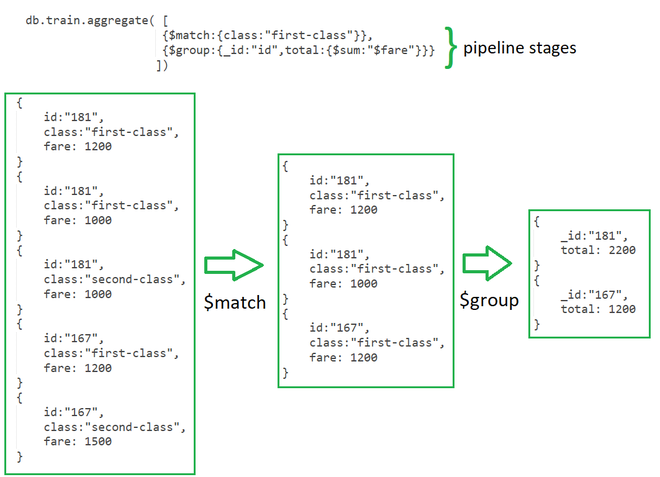In the above example of a collection of train fares in the first stage. Here, the \$match stage filters the documents by the value in class field i.e. class: “first-class” and passes the document to the second stage. In the Second Stage, the \$group stage groups the documents by the id field to calculate the sum of fare for each unique id.

Here, the aggregate() function is used to perform aggregation it can have three operators stages, expression and accumulator.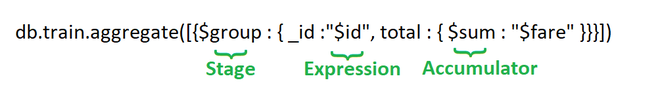Stages: Each stage starts from stage operators which are:

• \$match: It is used for filtering the documents can reduce the amount of documents that are given as input to the next stage.
• \$project: It is used to select some specific fields from a collection.
• \$group: It is used to group documents based on some value.
• \$sort: It is used to sort the document that is rearranging them
• \$skip: It is used to skip n number of documents and passes the remaining documents
• \$limit: It is used to pass first n number of documents thus limiting them.
• \$unwind: It is used to unwind documents that are using arrays i.e. it deconstructs an array field in the documents to return documents for each element.
• \$out: It is used to write resulting documents to a new collection

Expressions: It refers to the name of the field in input documents for e.g. { \$group : { _id : “\$id“, total:{\$sum:”\$fare“}}} here \$id and \$fare are expressions.

Accumulators: These are basically used in the group stage

• sum: It sums numeric values for the documents in each group
• count: It counts total numbers of documents
• avg: It calculates the average of all given values from all documents
• min: It gets the minimum value from all the documents
• max: It gets the maximum value from all the documents
• first: It gets the first document from the grouping
• last: It gets the last document from the grouping

Note:

• in \$group _id is Mandatory field
• \$out must be the last stage in the pipeline
• \$sum:1 will count the number of documents and \$sum:”\$fare” will give the sum of total fare generated per id.

Examples:

In the following examples, we are working with:

Database: GeeksForGeeks

Collection: students

Documents: Seven documents that contain the details of the students in the form of field-value pairs.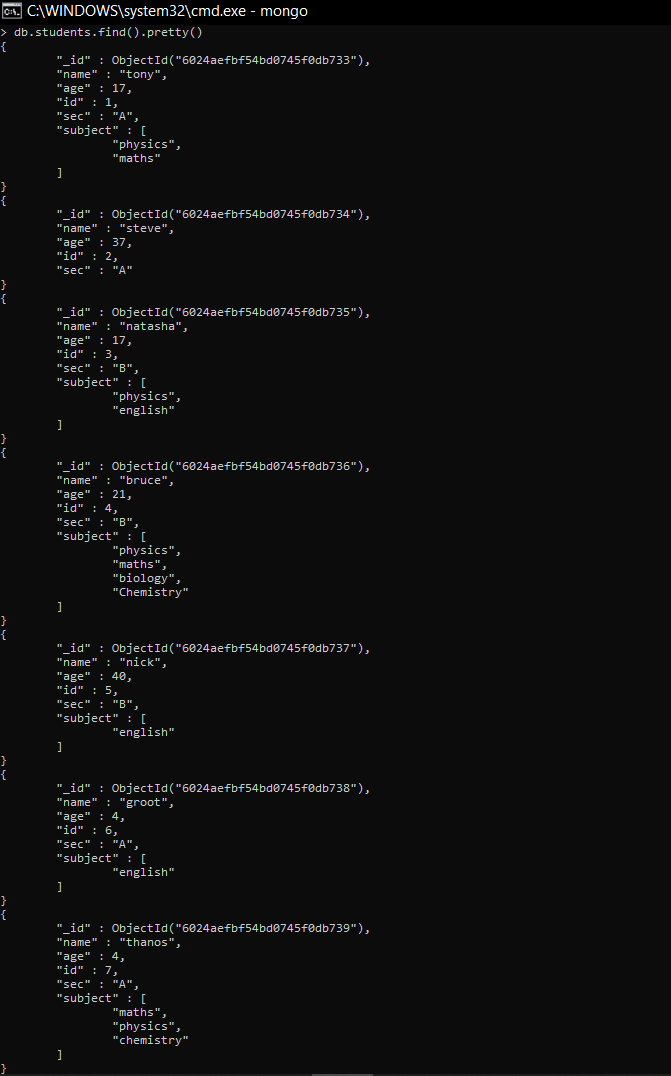• Displaying the total number of students in one section only
`db.students.aggregate([{\$match:{sec:"B"}},{\$count:"Total student in sec:B"}])`

In this example, for taking a count of the number of students in section B we first filter the documents using the \$match operator, and then we use the \$count accumulator to count the total number of documents that are passed after filtering from the \$match.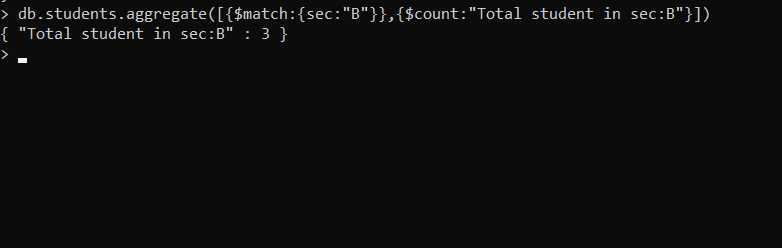• Displaying the total number of students in both the sections and maximum age from both section
`db.students.aggregate([{\$group: {_id:"\$sec", total_st: {\$sum:1}, max_age:{\$max:"\$age"} } }])`

In this example, we use \$group to group, so that we can count for every other section in the documents, here \$sum sums up the document in each group and \$max accumulator is applied on age expression which will find the maximum age in each document.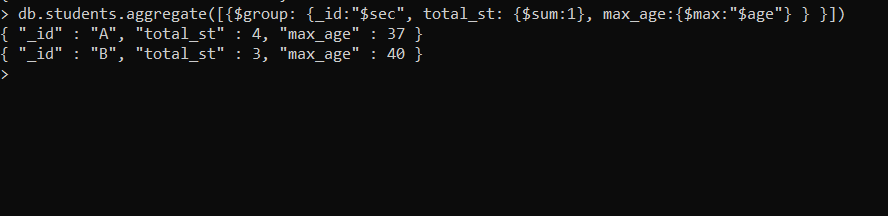• Displaying details of students whose age is greater than 30 using match stage
`db.students.aggregate([{\$match:{age:{\$gt:30}}}])`

In this example, we display students whose age is greater than 30. So we use the \$match operator to filter out the documents.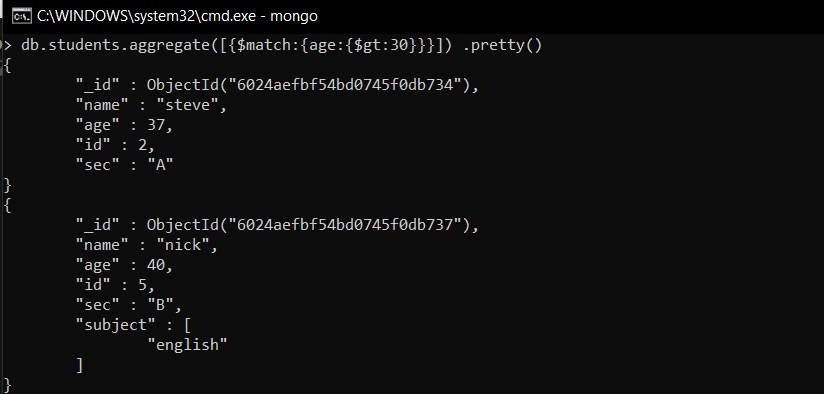• Sorting the students on the basis of age
`db.students.aggregate([{'\$sort': {'age': 1}}])`

In this example, we are using the \$sort operator to sort in ascending order we provide ‘age’:1 if we want to sort in descending order we can simply change 1 to -1 i.e. ‘age’:-1.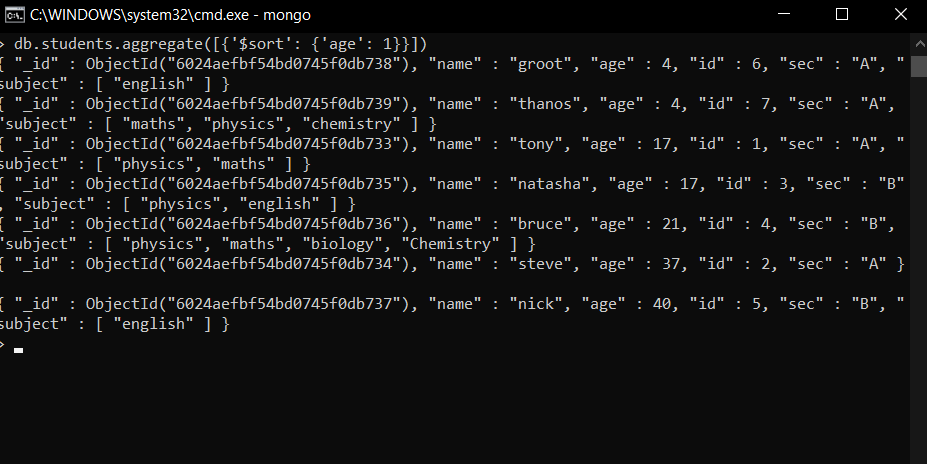• Displaying details of a student having the largest age in the section – B
`db.students.aggregate([{\$match:{sec:"B"}},{'\$sort': {'age': -1}},{\$limit:1}])`

In this example, first, we only select those documents that have section B, so for that, we use the \$match operator then we sort the documents in descending order using \$sort by setting ‘age’:-1 and then to only show the topmost result we use \$limit.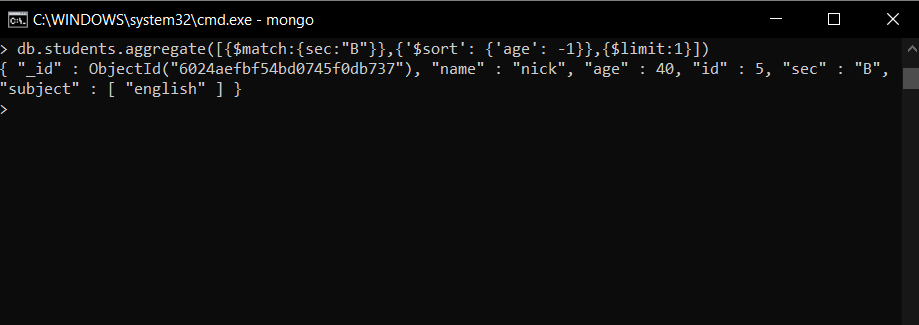• Unwinding students on the basis of subject

Unwinding works on array here in our collection we have array of subjects (which consists of different subjects inside it like math, physics, English, etc) so unwinding will be done on that i.e. the array will be deconstructed and the output will have only one subject not an array of subjects which were there earlier.

`db.students.aggregate([{\$unwind:"\$subject"}]) `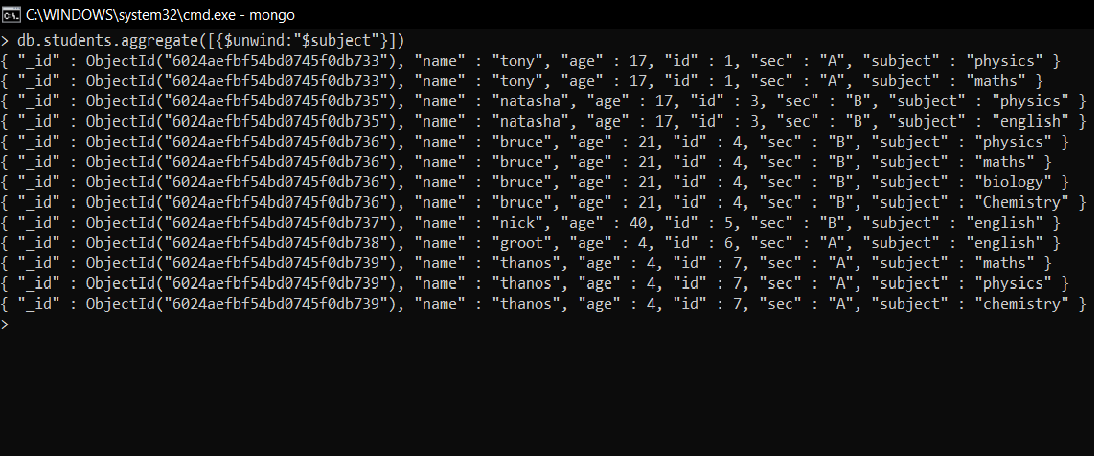## Map Reduce

Map reduce is used for aggregating results for the large volume of data. Map reduce has two main functions one is a map that groups all the documents and the second one is the reduce which performs operation on the grouped data.

Syntax:

`db.collectionName.mapReduce(mappingFunction, reduceFunction, {out:'Result'});`

Example:

In the following example, we are working with:

Database: GeeksForGeeks

Collection: studentsMark

Documents: Seven documents that contain the details of the students in the form of field-value pairs.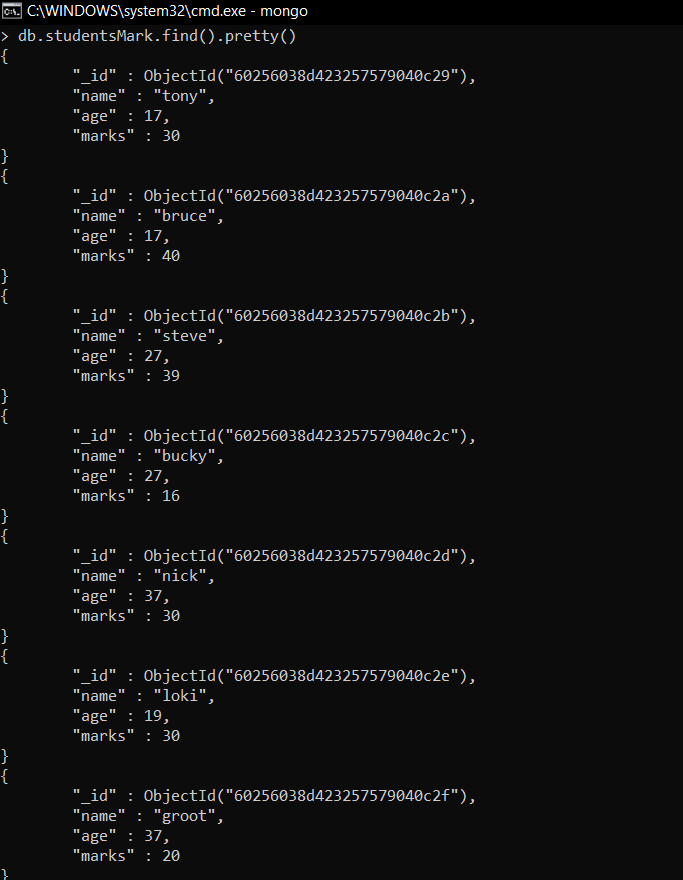```var mapfunction = function(){emit(this.age, this.marks)}
var reducefunction = function(key, values){return Array.sum(values)}
db.studentsMarks.mapReduce(mapfunction, reducefunction, {'out':'Result'})```

Now, we will group the documents on the basis of age and find total marks in each age group. So, we will create two variables first mapfunction which will emit age as a key (expressed as “_id” in the output) and marks as value this emitted data is passed to our reducefunction, which takes key and value as grouped data, and then it performs operations over it. After performing reduction the results are stored in a collection here in this case the collection is Results.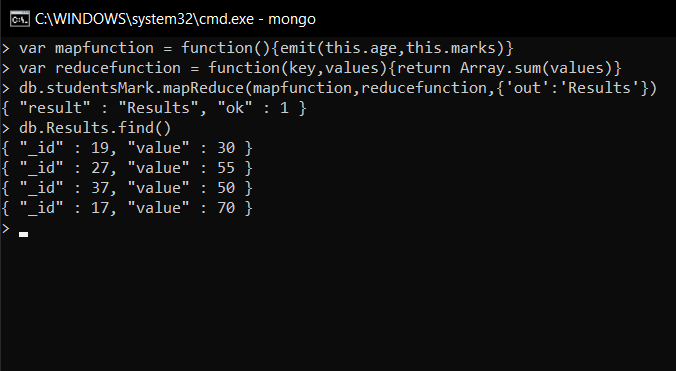## Single Purpose Aggregation

It is used when we need simple access to document like counting the number of documents or for finding all distinct values in a document. It simply provides the access to the common aggregation process using the count(), distinct(), and estimatedDocumentCount() methods, so due to which it lacks the flexibility and capabilities of the pipeline.

Example:

In the following example, we are working with:

Database: GeeksForGeeks

Collection: studentsMark

Documents: Seven documents that contain the details of the students in the form of field-value pairs.• Displaying distinct names and ages (non-repeating)
`db.studentsMarks.distinct("name")`

Here, we use a distinct() method that finds distinct values of the specified field(i.e., name).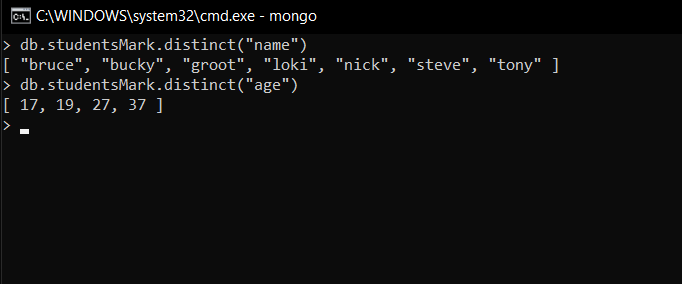• Counting the total numbers of documents
`db.studentsMarks.count()`

Here, we use count() to find the total number of the document, unlike find() method it does not find all the document rather it counts them and return a number.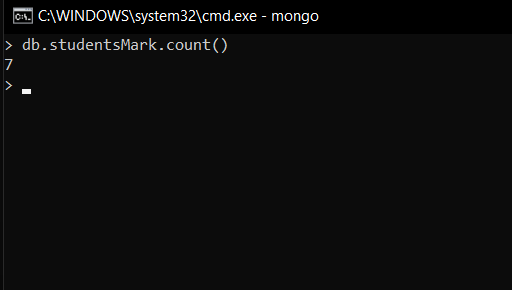My Personal Notes arrow_drop_up